# Rotate a Square Matrix by 90 Degrees in JavaScript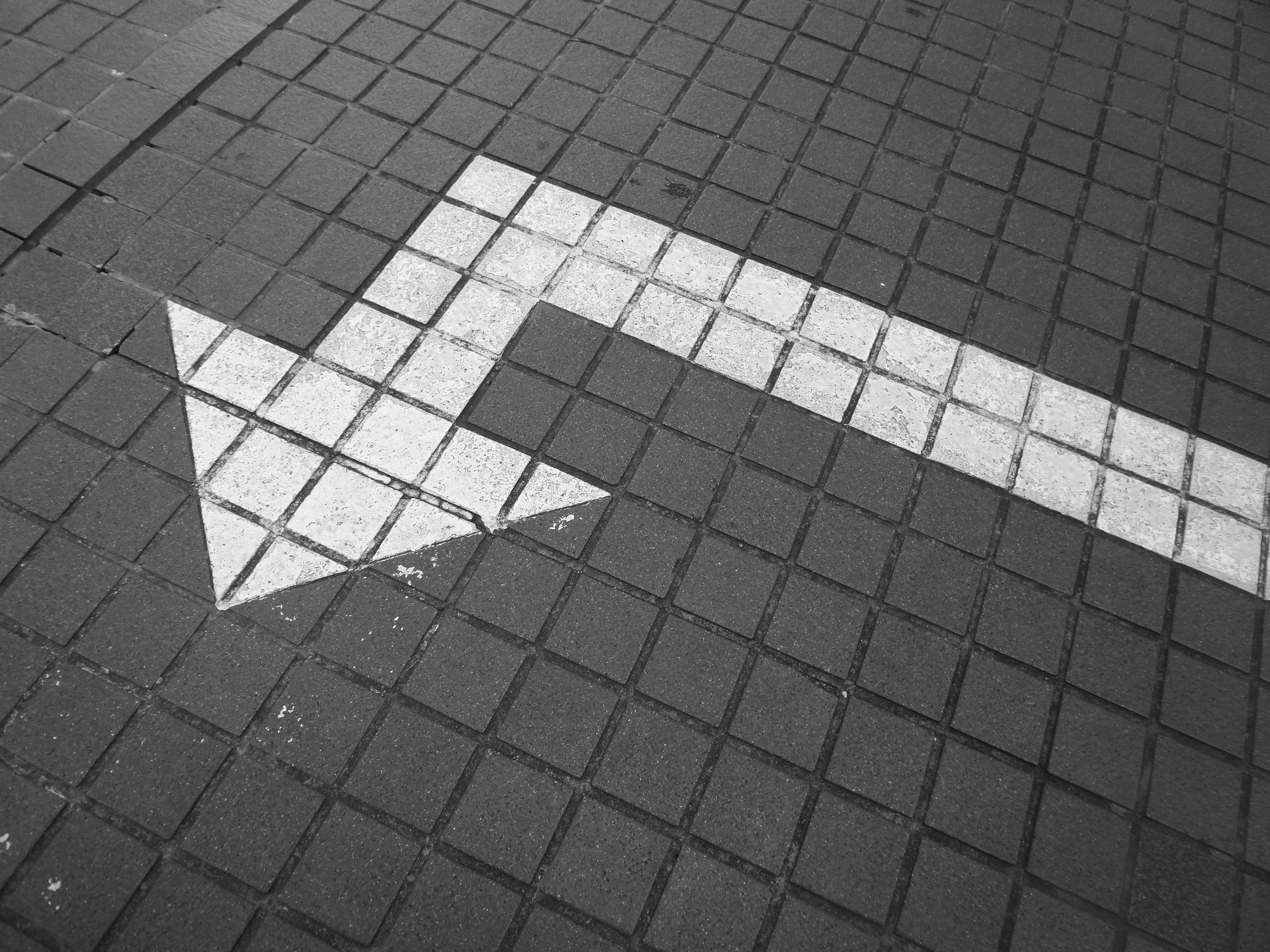Photo by Possessed Photography on Unsplash

In this blog I’m going to teach you one method for rotating a square matrix 90 degrees clockwise, in place. I came across this question on leetcode. I thought I would have trouble remembering the steps to solve this problem, so I wanted to write a blog about it. Hopefully in addition to helping me remember how to solve this problem, I can help others understand it as well.

# Step by Step Example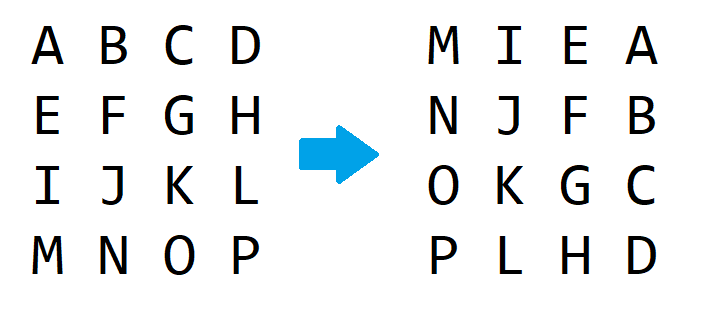matrix before and after 90 degree rotation

To rotate our matrix 90 degrees clockwise, we will work starting from the ‘outside’ and work towards the ‘center’. I will use images to hopefully make what I mean more clear as we go along.

## First Step

In our first step, we will swap all of the elements at the corners of the matrix so that they are in their correct location.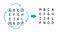matrix rotation step 1

## Second Step

Now we move over one element from each corner, and swap again.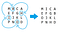matrix rotation step 2

## Third Step

Now we perform the last rotation on our outermost layer.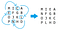matrix rotation step 3

As you can see only the center 4 elements are out of place now.

## Final Step

Now we only need to swap the last four elements in our matrix.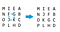matrix rotation final step (step 4)

Our matrix has been rotated 90 degrees clockwise! I found that the concept behind rotating the matrix wasn’t so difficult, but it was difficult figure out how to translate it into code.

# Code

To start our code we need to loop through our matrix. We will be keeping track of elements in the top left, top right, bottom left, and bottom right positions. Let’s define a while loop that will keep pointers to the left and right sides.

We also need some way to figure out our top and bottom positions, we can accomplish that by putting a for loop inside of our while loop.

For each iteration of the `for` loop, we need to:

• Swap the bottom left into the top left
• Swap bottom right into bottom left
• Swap top right into bottom right
• Swap the top left into top right

These swaps are just like our step by step example. Because we are dealing with a square matrix, the top value will be equal to left, and the bottom will be equal to right. Because of this, there is no need to declare any additional variables but I think that it helps to clarify the code.

Now it’s time to actually swap the elements in the matrix!

One of the more difficult things for me to understand was adding and subtracting `i`. What helped me understand was to think about what happened after swapping the 4 elements.

• Top left moved to the right by one (left + i)
• Top right moved down by one (top + i)
• Bottom right moved left by one (right - i)
• Bottom left moved up by one (bottom - i)

The other thing that was difficult for me to grasp was the inner `for` loop. The easiest way for me to explain it is that, we are going layer by layer. As we go one layer deeper, we need to do 2 less rounds of swaps, and start from 1 element deeper (hence why we start from left, which keeps incrementing). In our step by step example, we do 3 rounds of swaps on the outer layer, and only 1 on the inner layer. If we had a 10 * 10 matrix, we would do 9 swaps, then 7, then 5, then 3, and then 1.

# Time and Space Complexity

## Time Complexity

The time complexity of this function is O(m) where m represents the number of elements in the matrix. This is because each element is getting read and moved once before it arrives in its correct position.

## Space Complexity

Since we are rotating the matrix in place, the space complexity is O(1).

# In Conclusion…

I hope this blog post helped you to understand this way of rotating a matrix by 90 degrees. Understanding the concept of where to move the elements isn’t so difficult, but it proves to be one of those things that is difficult translate into code. The code for this function can be found in this GitHub repository.

Aspiring Software Engineer

## More from Regina Furness

Aspiring Software Engineer